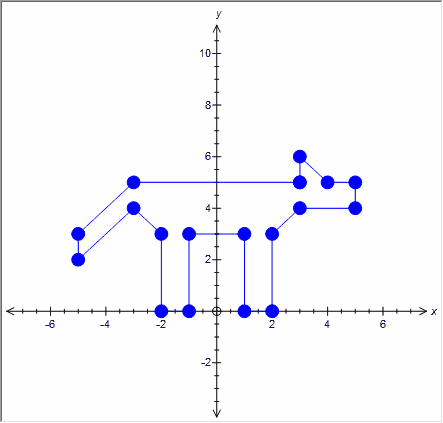﻿ Groups of Points

# Groups of Points

Top  Previous  Next
 The graph tool can plot groups of points and join them together.  Simply place a + sign between the points. See regional issues.   (3,5)+(3,6)+(4,5)+(5,5)+(5,4)+(3,4)+(2,3)+(2,0)+(1,0)+(1,3)+(-1,3)+(-1,0)+(-2,0)+(-2,3)+(-3,4)+(-5,2)+(-5,3)+(-3,5)+(3,5)You can mix points and groups of points in the same function.   Regional Issues   If you use a comma as a decimal point (eg 3,2) you must use a semicolon to separate the two coordinates.   (3;5)+(3;6)+(4;5)+(5;5)+(5;4)+(3;4)+(2;3)+(2;0)+(1;0)+(1;3)+(-1;3)+(-1;0)+(-2;0)+(-2;3)+(-3;4)+(-5;2)+(-5;3)+(-3;5)+(3;5)